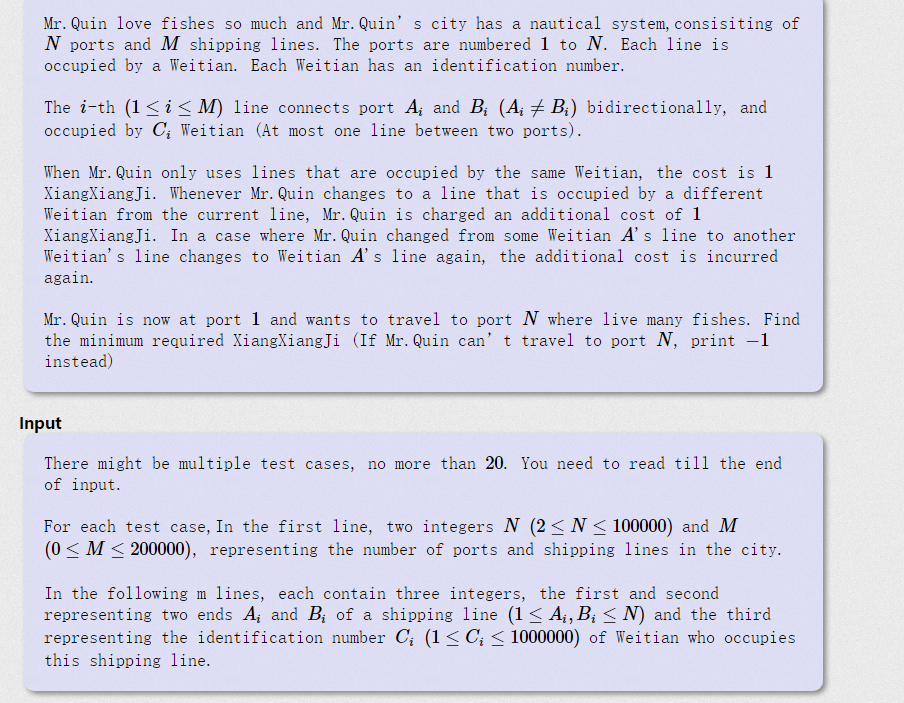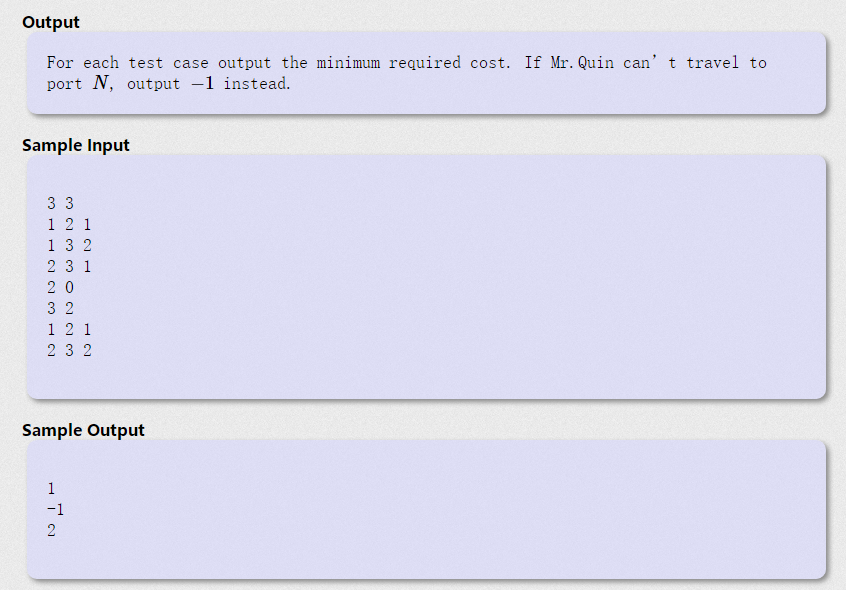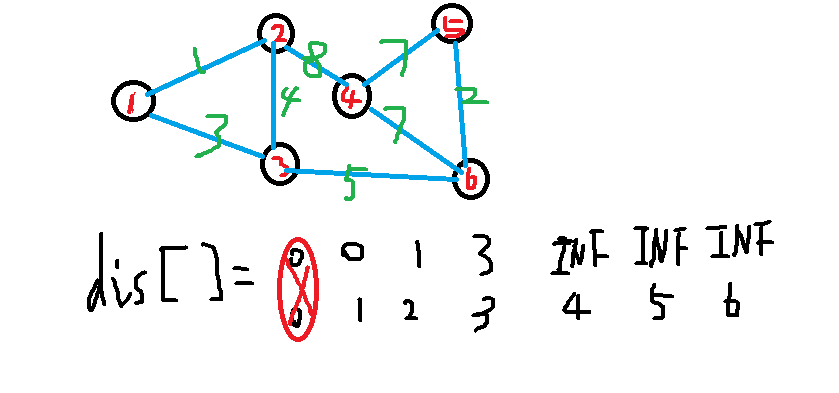## A - Age of Moyu HDU - 6386（Dijkstra+堆优化）Dijkstra思想：我先利用dis[]数组来存下1能到达的所有点的距离，然后其他点置为INF（通常INF令为0x3f3f3f3f就够了）；``````#include<bits/stdc++.h>
using namespace std;
typedef long long ll;
#define INF 0x3f3f3f3f
int Map,n,m;//Map用来记录a点到b点的路径值，n表示有多少个点,m表示给出多少条边
int dis,book;//dis是用来表示 start点到其他点的距离，book用来标记 循环到这个点的是否被标记过
void dijkstra(int start){//最简单的dijkstra算法（采用矩阵形式）
//首先求出这个点到其他点的距离用一个for
int Min=INF;
for(int i=1;i<=n;i++){//初始化从start这点开始的所有能与start点可达的点的距离
dis[i]=Map[start][i];//注意这里dis和Map只是一个逻辑关系相联系
}
//这里需要枚举n-1个点，因为开始点不需要算
//所以用一个大的for来循环
book[start]=1;//标记开始点已经作为中转站过了
for(int i=1;i<=n-1;i++){
//找出第一个到start的最短的点
//并且标记已经选过了
Min=INF;
int u;//记录到start最短的点
for(int j=1;j<=n;j++){
if(book[j]==0&&dis[j]<Min){//去搜寻能与start有直接边的点的最小距离//这里最好头脑里面想着无向图
Min=dis[j];
u=j;
}
}
book[u]=1;//表示u这个点已经作为中转站了
//然后找到以u点为中转站的其他点到start的距离和经过u这个点的距离，并比较大小
for(int e=1;e<=n;e++){//这里e是end的简写，方便理解表示以u作为中转站所能到达的另一个点
if(Map[u][e]<INF){
if(dis[e]>dis[u]+Map[u][e]){//这就是上面分析的比较
dis[e]=dis[u]+Map[u][e];
}
}
}
}
}
void init(int n){
for(int i=1;i<=n;i++)
{
for(int j=1;j<=n;j++){
if(i==j) Map[i][j]=0;//自己到自己就是0
else Map[i][j]=INF;//其他的设为INF
}
}
}
{
int a,b,c;
for(int i=1;i<=m;i++){
cin>>a>>b>>c;
Map[a][b]=c;//令为无向图
Map[b][a]=c;
}
}
int main(){
cin>>n>>m;
init(n);//这里虽然n，m为全局变量，但是这样写参数传递更加好理解；
dijkstra(1);//从第一个点开始（当然这里也可以从别的点开始）
for(int i=2;i<=n;i++){//输出到其他点的最短距离
cout<<"1到"<<i<<"的最短距离为："<<dis[i]<<"\n";
}
cout<<endl;
return 0;
}
``````

``````#include<bits/stdc++.h>
using namespace std;
#define INF 0x3f3f3f3f
typedef long long ll;
ll n,m;
struct End_Dis{
ll to,d,c;//to表示到的点，d表示到这个兵兵（也可以理解为距离问题）,c用来记录是不是有这个兵兵在这条路上
End_Dis(ll a,ll b,ll cc=0):to(a),d(b),c(cc){}
bool operator < (const End_Dis tt) const{
return d>tt.d;//这个比较特殊，因为优先队列是由大到小，所以我写了一个由大到小的，然后这样会让优先队列由小到大排列（特别的地方）
}
};
vector<End_Dis> a[100000*2];
ll book1;//用来标记这个点扫过没有
ll Dijkstra(ll start){
ll dis;
for(ll i=1;i<=n;i++) dis[i]=INF;//先初始化为INF
book1=0;//表示这个点走过了
dis=0;//自己到自己距离为0；
priority_queue<End_Dis> q;//建立一个优先队列
q.push(End_Dis(1,0));//把第一个点放进去
while(!q.empty()) {//循环为非空
End_Dis t=q.top();
q.pop();
if(book1[t.to]) continue;//表示这个点使用过没有
for(ll i=0;i<a[t.to].size();i++){//以这个点位中转站，枚举其他点更新1----其他点的最短距离
End_Dis x=a[t.to][i];//取出对象
if((t.c!=x.d)+t.d<dis[x.to]) {//1到中转站h的距离+前面是否出现过的值是否小于  1---x的距离
dis[x.to]=(t.c!=x.d)+t.d;
q.push(End_Dis(x.to,dis[x.to],x.d));//找到了之后然后让中转站后面的点进队
}
}
}
return dis[n];
}
ll aa,bb,cc;
for(ll i=1;i<=m;i++){
scanf("%lld %lld %lld",&aa,&bb,&cc);
a[aa].push_back(End_Dis(bb,cc));
a[bb].push_back(End_Dis(aa,cc));
}
}
void init(ll n){
for(ll i=1;i<=n;i++){
a[i].clear();
}
}
int main(){
while(~scanf("%lld %lld",&n,&m)){
init(n);
int ans=Dijkstra(1);
if(ans==INF)puts("-1");
else printf("%lld\n",ans);
}
return 0;
}
``````

``````#include<bits/stdc++.h>
using namespace std;
#define INF 0x3f3f3f3f
typedef long long ll;
ll n,m;
struct End_Dis{
ll to,d;//to表示到的点，d表示为前一个点到to这个点的距离
End_Dis(ll a,ll b):to(a),d(b){}///这里构造函数有便于加点
bool operator < (const End_Dis tt) const{//这里在使用优先队列的时候很有用
return d>tt.d;//这个比较特殊，因为优先队列是由大到小，所以我写了一个由大到小的，然后这样会让优先队列由小到大排列（特别的地方）
}
};
vector<End_Dis> a[100000*2];//用邻接表来存储一个点能到的其他点比如：a[aa][i].to表示aa这个点到to这个点
ll book1;//用来标记这个点扫过没有//用来标记这个点是否作为过中转站
ll Dijkstra(ll start){
ll dis;//表示距离
for(ll i=1;i<=n;i++) dis[i]=INF;//先初始化为INF
book1[start]=0;//表示这个点走过了//这里需要注意了 因为这里是和最简单的Dijkstra算法不同点
dis[start]=0;//自己到自己距离为0；
priority_queue<End_Dis> q;//建立一个优先队列
q.push(End_Dis(start,0));//把第一个点放进去
while(!q.empty()) {//循环为非空
End_Dis t=q.top();
q.pop();
if(book1[t.to]) continue;//表示这个点使用过没有
for(ll i=0;i<a[t.to].size();i++){//以这个点位中转站，枚举其他点更新1----其他点的最短距离
End_Dis x=a[t.to][i];//取出t.to这个点所能到达的点的对象
if(dis[x.to]>dis[t.to]+x.d){//start到x.to点的距离 和   start到t.to的距离与t.to到x.d的和的比较
//更新这个点的距离
dis[x.to]=dis[t.to]+x.d;
q.push(End_Dis(x.to,dis[x.to]));//把这个点加入优先队列中
}
}
}
return dis[n];
}
ll aa,bb,cc;
for(ll i=1;i<=m;i++){
scanf("%lld %lld %lld",&aa,&bb,&cc);
a[aa].push_back(End_Dis(bb,cc));
a[bb].push_back(End_Dis(aa,cc));
}
}
void init(ll n){//每次清空
for(ll i=1;i<=n;i++){
a[i].clear();
}
}
int main(){
while(~scanf("%lld %lld",&n,&m)){
init(n);
int ans=Dijkstra(1);
if(ans==INF)puts("-1");//如果不能到n点那么久输出-1
else printf("到n点的最短路径为：   %lld\n",ans);
}
return 0;
}
``````